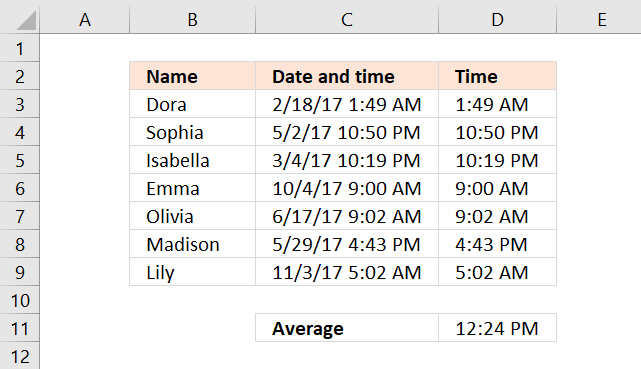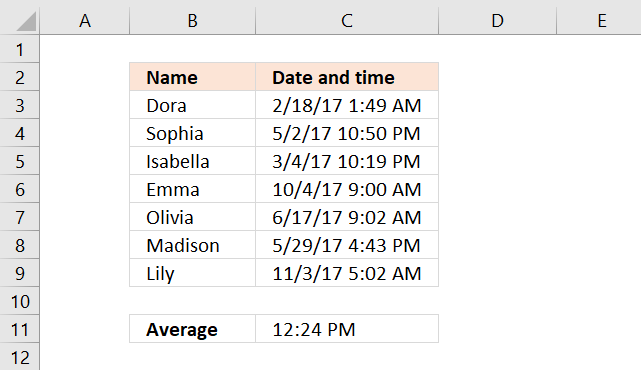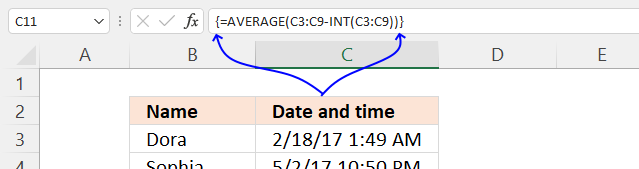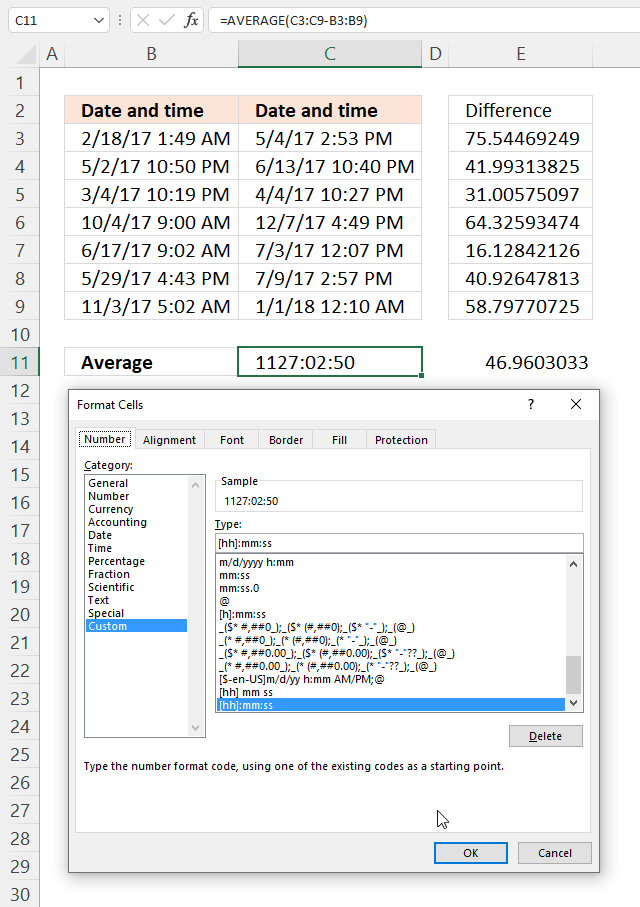Author: Oscar Cronquist Article last updated on December 15, 2021## 1. How to AVERAGE timeThe formula demonstrated in cell C11 calculates the average time based on the time values in cell range C3:C9. It doesn't take the date part into the calculations.

=AVERAGE(C3:C9 -INT(C3:C9))

### 1.1 How to enter an array formula

You need to enter the formula as an array formula if you use an earlier version than Excel 365.

The formula above is an array formula. To enter an array formula, type the formula in a cell then press and hold CTRL + SHIFT simultaneously, now press Enter once. Release all keys.The formula bar now shows the formula enclosed with curly brackets telling you that you entered the formula successfully. Don't enter the curly brackets yourself.

### 1.2 Explaining formula

An Excel date and time value is in fact a number and a decimal part formatted as a date. For example, number 1 is 1/1/1900 and 1.5 is 1/1/1900 12:00 PM. This makes it really easy to add or subtract dates in Excel.

#### Step 1 - Extract whole numbers

The INT function returns the integer part of a number.

INT(number)

INT(C3:C9)

becomes

INT({42784.0758894521; 42857.9518970912; 42798.9302136859; 43012.3751426585; 42903.3770360669; 42884.6971055089; 43042.2098422595})

and returns

{42784; 42857; 42798; 43012; 42903; 42884; 43042}.

Notice how the values lost their decimal part, only the whole numbers remains.

#### Step 2 - Extract decimals

The date part is the whole number and the decimals are the times, we can extract the time part by subtracting the original numbers with the whole numbers.

The minus sign lets you subtract a number with another number in Excel formulas.

C3:C9 -INT(C3:C9)

becomes

{42784.0758894521; 42857.9518970912; 42798.9302136859; 43012.3751426585; 42903.3770360669; 42884.6971055089; 43042.2098422595} - {42784; 42857; 42798; 43012; 42903; 42884; 43042}

and returns

{0.0758894520549802; 0.951897091246792;0.930213685918716; 0.37514265847858; 0.377036066871369; 0.69710550889431; 0.209842259464494}

#### Step 3 - Average decmial numbers

The AVERAGE function calculates an average.

AVERAGE(number1[number2], ...)

AVERAGE(C3:C9 -INT(C3:C9))

becomes

AVERAGE({0.0758894520549802; 0.951897091246792;0.930213685918716; 0.37514265847858; 0.377036066871369; 0.69710550889431; 0.209842259464494})

and returns 0.516732388989892.

## 2. How to AVERAGE time hh mm ssThe formula in cell C11 calculates an average based on the differences of the Excel dates and time values in columns B and C.

Formula in cell C11:

=AVERAGE(C3:C9-B3:B9)

Cell formatting is applied to cell C11:

1. Select cell C11.
2. Press CTRL + 1 to open the "Format Cells" dialog box, see the image above.
3. Press with left mouse button on "Custom" category.
4. Enter a new "type": [hh]:mm:ss
5. Press with left mouse button on OK button.

### 2.1 Explaining formula

An Excel date and time value is in fact a number and a decimal part formatted as a date. For example, number 1 is 1/1/1900 and 1.5 is 1/1/1900 12:00 PM. This makes it really easy to add or subtract dates in Excel.

#### Step 1 - Calculate difference between date and time values

The minus sign lets you subtract a number with another number in Excel formulas.

C3:C9-B3:B9

becomes

{42859.6205819411; 42899.94503534; 42829.9359646512; 43076.7010774007; 42919.5054573317; 42925.6235836372; 43101.0075495109}-{42784.0758894521; 42857.9518970912; 42798.9302136859; 43012.3751426585; 42903.3770360669; 42884.6971055089; 43042.2098422595}

and returns

{75.5446924890348; 41.9931382487848; 31.0057509652324; 64.3259347422427; 16.1284212648752; 40.9264781282691; 58.7977072514477}.

#### Step 2 - Calculate the average

The AVERAGE function calculates an average.

AVERAGE(number1[number2], ...)

AVERAGE(C3:C9-B3:B9)

becomes

AVERAGE({75.5446924890348; 41.9931382487848; 31.0057509652324; 64.3259347422427; 16.1284212648752; 40.9264781282691; 58.7977072514477})

and returns approx. 46.96 in cell C11, however, the value is formatted to show total hours, seconds and milliseconds.

## Get Excel *.xlsx file

How to AVERAGE time.xlsx